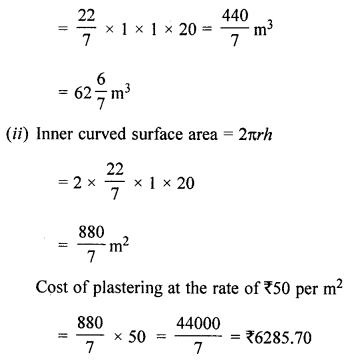## ML Aggarwal Class 8 Solutions for ICSE Maths Chapter 18 Mensuration Check Your Progress

Question 1.
A square field of side 65 m and rectangular field of length 75 m have the same perimeter. Which field has a larger area and by how much?
Solution:
Side of a square field = 65 m
∴ Perimeter = 4 × Side = 4 × 65 = 260 m
Now perimeter of a rectangular field = 260 m
and length = 75 m
Perimeter of rectangle = 2(l + B)
∴ Breadth = $$\frac{P}{2}-l=\frac{260}{2}$$ – 75 = 130 – 75 = 55 m
Area of square field = (side)2 = (65)2 m= 4225 m2
and area of rectangular field = l × b = 75 × 55 m = 4125 m2
It is clear that area of square field is greater difference = 4225 – 4125 = 100 m2

Question 2.
The shape of a top surface of table is a trapezium. Find the area if its parallel sides are 1.5 m and 2.5 m and perpendicular distance between them is 0.8 m.
Solution:
Shape of the top of a table is trapezium and parallel sides are
1.5 m and 2.5 m and the perpendicular distance between then = 0.8m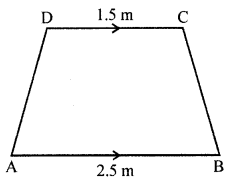Area = $$\frac{1}{2}$$ (Sum of parallel sides) × height
= $$\frac{1}{2}$$(1.5 + 2.5) × 0.8
= $$\frac{1}{2}$$ × 4 × 0.8 m2 = 1.6 m2

Question 3.
The length and breadth of a hall of a school are 26 m and 22 m respectively. If one student requires 1.1 sq. m area, then find the maximum number of students to be seated in this hall.
Solution:
Length of a school hall (l) = 26 m
and breadth (b) = 22 m
∴ Area = l × b = 26 × 22 m2 = 572 m2
One student requires 1.1 sq. m area
∴ Number of students = $$\frac{572}{1.1}$$
= $$\frac{572 \times 10}{11}$$ = 520 students

Question 4.
It costs ₹936 to fence a square field at ₹7·80 per metre. Find the cost of levelling the field at ₹2.50 per square metre.
Solution:
Cost of fencing the square field at ₹7·80 per metre = ₹936.
∴ Total fence required = $$\frac{936}{7 \cdot 80}$$ = 120
⇒ Perimeter of the field = 120 m
⇒ 4 × Side = 120 m (∵ Field is square)
Side = $$\frac{120}{4}$$
∴ Side = 30 m
Hence, Area of square field
= (30)2 = 900 m2
= 900 × 2.50 = ₹2250

Question 5.
Find the area of the shaded portion in the following figures all measurements are given in cm.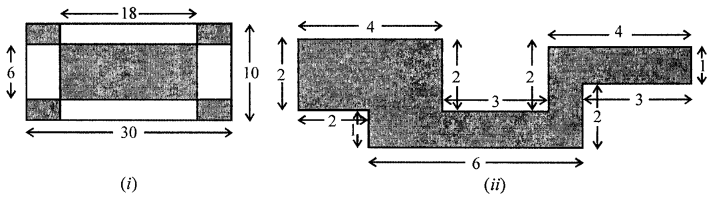Solution:
(i) Outer length = 30 cm
Side of each rectangle of the corner (l)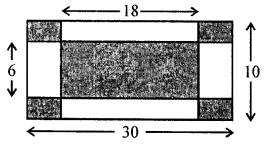= $$\frac{30-18}{2}=\frac{12}{2}$$ = 6 cm
and b = 10 – 6 = $$\frac{4}{2}$$ = 2 cm
∴ Area of 4 comer = 6 × 2 × 4 = 48 cm2
and area of inner rectangle = 18 × 6 = 108 cm2
∴ Area of shaded portion = 108 + 48 = 156cm2
(ii) Area of rectangle I = 4 × 2 = 8 cm2
Area of rectangle II = 4 × 1 = 4 cm2
Area of rectangle III = 6 × l = 6 cm2
and area of square IV = 1 × 1 = 1 Cm2
∴ Total area of shaded portion = 8 + 4 + 6 + 1 = 19 cm2Question 6.
Area of a trapezium is 160 sq. cm. Lengths of parallel sides are in the ratio 1:3. If smaller of the parallel sides is 10 cm in length, then find the perpendicular distance between them.
Solution:
Area of trapezium = 160 cm2
Rate in length of parallel sides = 1 : 3
Smaller paralell side = 10 cm
Then length of greater side = $$\frac{10 \times 3}{1}$$ = 30 cm
Now, distance between them = h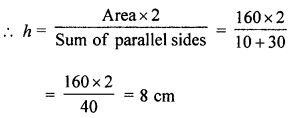Question 7.
The area of a trapezium is 729 cm2 and the distance between two parallel sides is 18 cm. If one of its parallel sides is 3 cm shorter than the other parallel side, find the lengths of its parallel sides.
Solution:
Area of a trapezium = 729 cm2
Distance between two parallel sides (Altitude) = 18 cm
∴ Sum of parallel sides = $$\frac{\text { Area } \times 2}{\text { Altitude }}$$
= $$\frac{729 \times 2}{18}$$ = 81 cm
One parallel side is shorter than the second by 3 cm
Let longer side = x
Then shorter side = x – 3
∴ x + x – 3 = 81
⇒ 2x = 81 + 3 = 84
x = $$\frac{84}{2}$$ = 42
∴ Longer side = 42 cm
and shorter side = 42 – 3 = 39 cm

Question 8.
Find the area of the polygon given in the figure: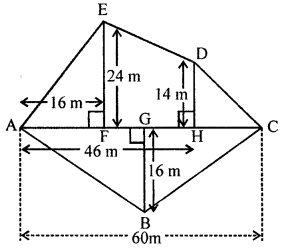Solution:
In the given figure,
AC = 60 m, AH = 46 m, AF = 16 m, EF = 24 m,
DH = 14 m, BG = 16 m
∴ FH = AH – AF = 46 – 16 = 30
HC = AC – AH = 60 – 46 = 14
In the figure, there are 3 triangles and one trapezium.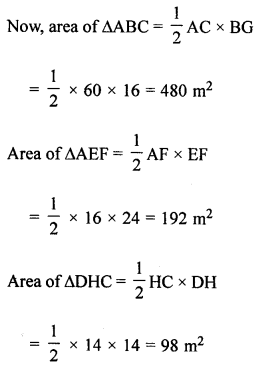Area of trapezium EFHD
= $$\frac{1}{2}$$(EF + DH) × FH
= $$\frac{1}{2}$$(24 + 14) × 30
= $$\frac{1}{2}$$ × 38 × 30 = 570 m2
∴ Total area of the figure,
= Area of ∆ABC + area ∆AEF + area ∆DHC + area trapezium EFHD
= 480 + 192 + 98 + 570 = 1340 m2

Question 9.
The diagonals of a rhombus are 16 m and 12 m, find:
(i) its area
(ii) length of a side
(iii) perimeter.
Solution:
Diagonals of a rhombus are d1 = 16 cm
and d2 = 12 cm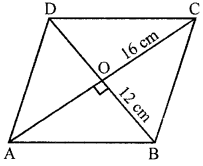(i) Area = $$\frac{d_{1} \times d_{2}}{2}=\frac{16 \times 12}{2}=96 \mathrm{cm}^{2}$$
(ii) ∵ Diagonals of rhombus bisect each other at right angles
∴ AO = OC = and BO = OD
AO = $$\frac{16}{2}$$ = 8 cm and BO = $$\frac{12}{2}$$ = 6 cm
Now in right ∆AOB
AB2 = AO2 + BO2 (Pythagoras Theorem)
= 82 + 62 = 64 + 36
= 100 = (10)2
∴ AB = 10 cm
∴ Side of rhombus = 10 cm

Question 10.
The area of a parallelogram is 98 cm2. If one altitude is half the corresponding base, determine the base and the altitude of the parallelogram.
Solution:
Area of a parallelogram = 98 cm2
One altitude = $$\frac{1}{2}$$ of its corresponding base
Let base = x cm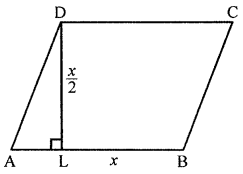Then altitude = $$\frac{x}{2}$$cm
∴ Area = Base × Altitude
98 = x × $$\frac{1}{2}$$x
⇒ x2 = 98 × 2 = 196 = (14)2
∴ x = 14
∴ Base = 14 cm and altitude = $$\frac{x}{2}=\frac{14}{2}$$ = 7 cm

Question 11.
Preeti is painting the walls and ceiling of a hall whose dimensions are 18 m × 15 m × 5 m. From each can of paint 120 m2 of area is painted. How many cans of paint does she need to paint the hall?
Solution:
Length of a hall (l) = 18 m
height (h) = 5 m
Area of 4-wall and ceiling = 2(l + b)h + lb
= 2(18 + 15) × 5 + 18 × 15 m2
= 2 × 33 × 5 + 270
= 330 + 270 = 600 m2
For paint of 120 m2 area, can of paint is required = 1
∴ Total number of cans required to paint the area of 600 m2 = $$\frac{600}{120}$$ = 5 cans

Question 12.
A rectangular paper is size 22 cm × 14 cm is rolled to form a cylinder of height 14 cm, find the volume of the cylinder. (Take π = $$\frac{22}{7}$$)
Solution:
Length of a rectangular paper = 22 cm
By rolling it a cylinder is formed whose height is 14 cm
and circumference of the base = 22 cm
Circumference = 2πr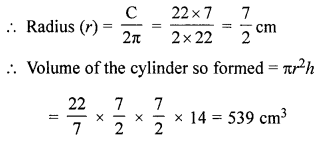Question 13.
A closed rectangular wooden box has inner dimensions 90 cm by 80 cm by 70 cm. Compute its capacity and the area of the tin foil needed to line its inner surface.
Solution:
Given that,
Inner length of rectangular box = 90 cm
Inner breadth of rectangular box = 80 cm
Inner height of rectangular box = 70 cm
Capacity of rectangular box = Volume of rectangular box
= l × b × h
= 90 cm × 80 cm × 70 cm = 504000 cm3
Required area of tin foil = 2 (lb + bh + lh)
= 2(90 × 80 + 80 × 70 + 90 × 70) cm2
= 2(7200 + 5600 + 6300) cm2
= 2 × 19100 cm2 = 38200 cm2

Question 14.
The lateral surface area of a cuboid is 224 cm2. Its height is 7 cm and the base is a square. Find
(i) side of the square base
(ii) the volume of the cuboid.
Solution:
Lateral surface area of a cuboid is 224 cm2
Height (h) = 7 cm
(i) 2(l + b) × h = 224
⇒ 2(l + b) × 7 = 224
l + b = $$\frac{224}{7 \times 2}$$ = 16 cm
But l = b (∵ The base of cuboid is a square)
∴ 2 × side = 16 cm
⇒ Side = $$\frac{16}{2}$$ = 8 cm
(ii) Volume of cuboid = lbh = 8 × 8 × 7 cm3 = 448 cm3

Question 15.
The inner dimensions of a closed wooden box are 2 m by 1.2 m by 0.75 m. The thickness of the wood is 2.5 cm. Find the cost of wood required to make the box if 1 m3 of wood costs ₹5400.
Solution:
Inner dimensioins of wooden box are 2 m, 1.2 m, 0.75 m
Thickness of the wood = 2.5 cm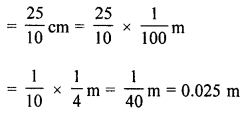External dimensions of wooden box are
(2 + 2 × 0.025), (1.2 + 2 × 0.025), (0.75 + 2 × 0.025)
= (2 + 0.05), (1.2 + 0.05), (0.75 + 0.5)
= 2.05, 1.25, 0.80
Volume of solid
= External volume of box – Internal volume of box
= 2.05 × 1.25 × 0.80 m3 – 2 × 1.2 × 0.75m3
= 2.05 – 1.80 = 0.25 m3
Cost = ₹5400 for 1 m3
Total cost = ₹5400 × 0.25 = ₹5400 × $$\frac{25}{100}$$
= ₹54 × 25 = ₹1350

Question 16.
A car has a petrol tank 40 cm long, 28 cm wide and 25 cm deep. If the ful consumption of the car averages 13.5 km per litre, how far can the car travel with a full tank of petrol?
Solution:
Capacity of car tank = 40 cm × 28 cm × 25 cm = (40 × 28 × 25) cm3
= $$\frac{40 \times 28 \times 25}{1000}$$ litre (∵ 1000 cm3 = 1 litre)
Average of car = 13.5 km per litres
Then, distance travelled by car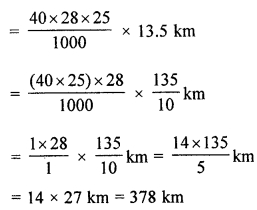Hence, the car can travel 378 km with a full tank of petrol.

Question 17.
The diameter of a garden roller is 1.4 m and it is 2 m long. How much area it will cover in 5 revolutions?
Solution:
Diameter of a garden rolle = 1.4 m
∴ Radius (r) = $$\frac{1.4}{2}$$ = 0.7 m = 70 cm
and length (h) = 2m
∴ Curved surface area = 2πrh
= 2 × $$\frac{22}{7}$$ × 70 × 200 cm2
= 88000 cm2
∴ Area covered in 5 revolutions = $$\frac{88000 \times 5}{10000}$$ m2 = 44 m2

Question 18.
The capacity of an open cylindrical tank is 2079 m3 and the diameter of its base is 21m. Find the cost of plastering its inner surface at ₹40 per square metre.
Solution:
Capacity of an open cylindrical tank = 2079 m3
Diameter of base = 21 m
∴ Radius (r) = $$\frac{21}{2}$$ m
Let h be the height, thenCost of plastering the surface = ₹40 × 742.5 = ₹29700

Question 19.
A solid right circular cylinder of height 1.21 m and diameter 28 cm is melted and recast into 7 equal solid cubes. Find the edge of each cube.
Solution:
Height of solid right circular cylinder = 1.21 m = 121 cm
and diameter = 28 cm
∴ Radius (r) = $$\frac{28}{2}$$ = 14 cm
Volume of the metal used = πr2h
= $$\frac{22}{2}$$ × 14 × 14 × 121 cm3
= 74536 cm3
∴ Volume of 7 solid cubes = 74536 cm3
Volume of 1 cube = $$\frac{74536}{7}$$ = 10648 cm3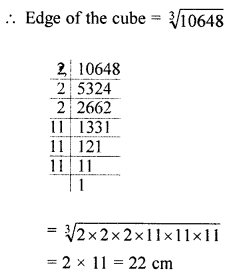Question 20.
(i) How many cubic metres of soil must be dug out to make a well 20 m deep and 2 m in diameter?
(ii) If the inner curved surface of the well in part (i) above is to be plastered at the rate of ₹50 per m2, find the cost of plastering.
Solution:
(i) Depth of a well (h) = 20 m
and diameter = 2 m
Radius (r) = $$\frac{2}{2}$$ = 1 m
Volume of earth dug out = πr2h